# 完美匹配：降压变换器功耗以及如何提高效率## 降压变换器功耗与效率基本原理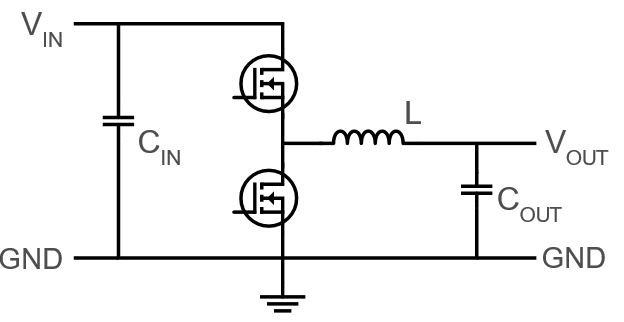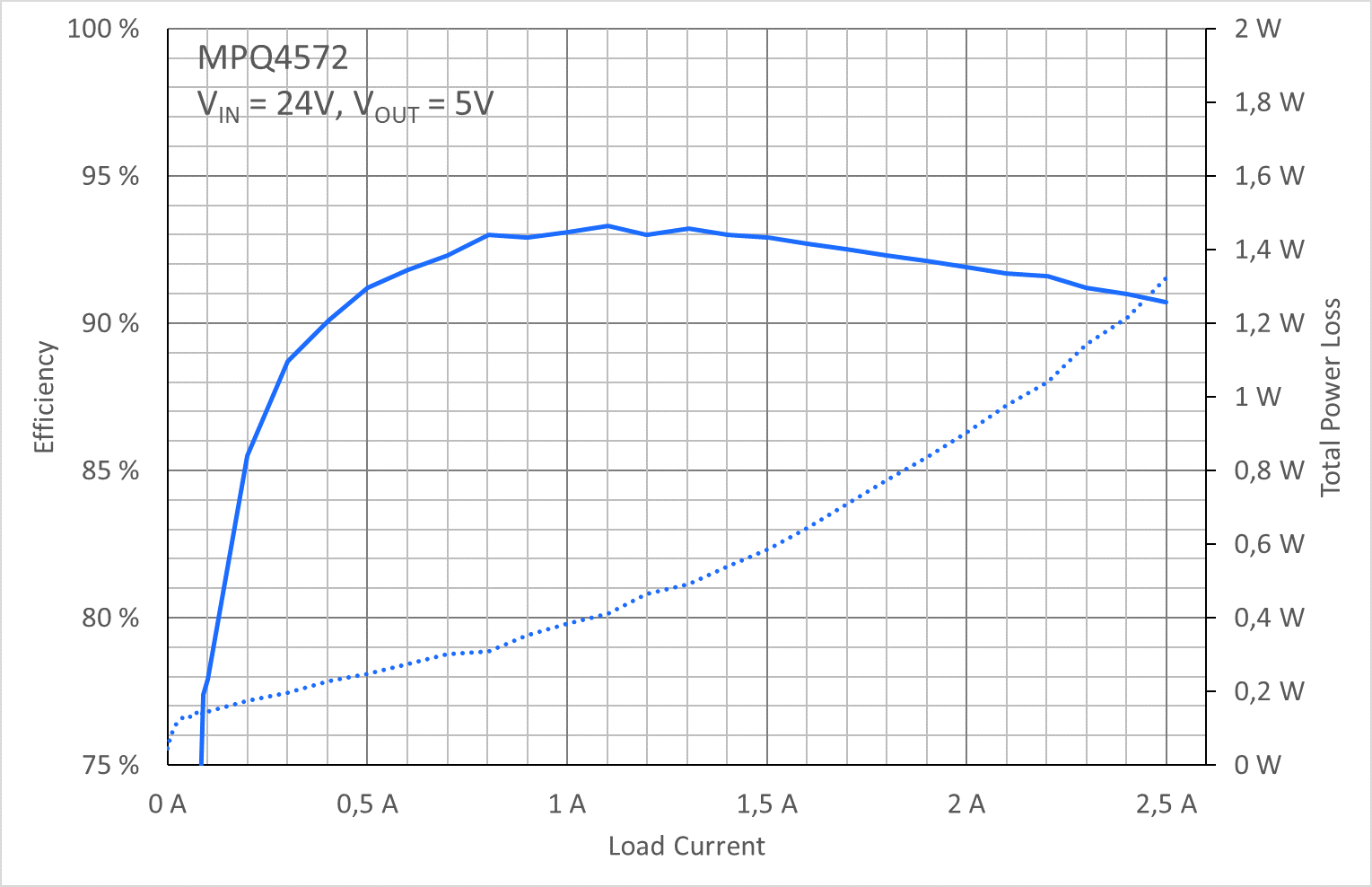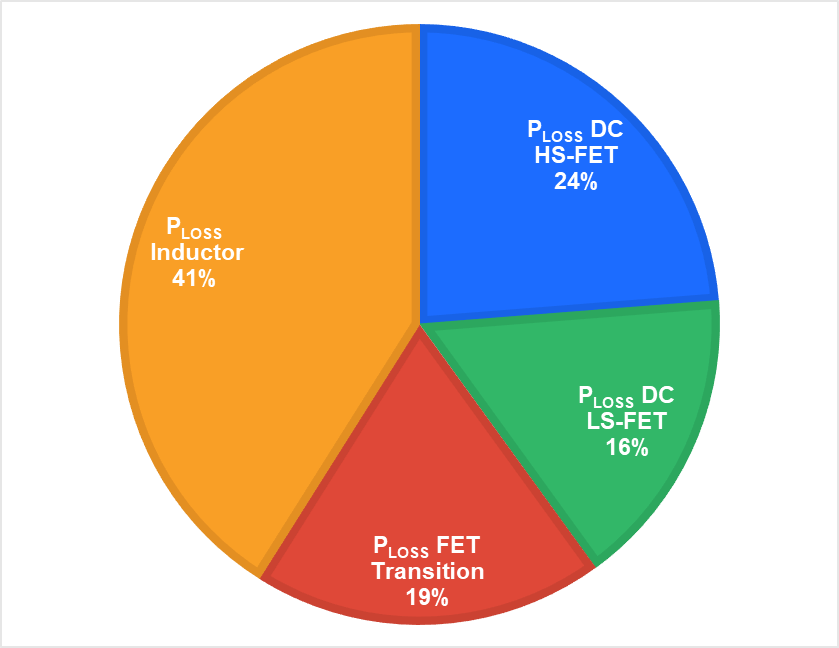## 电感量(L)

$$L = (1-DC) \times \left(\frac {V_{OUT}} {f_{sw \times \Delta I_{L}}}\right)$$

## 纹波电流(∆IL )

$$\Delta I_{L} = (1-DC) \left(\frac{V_{OUT}}^{\times L}}\right)$$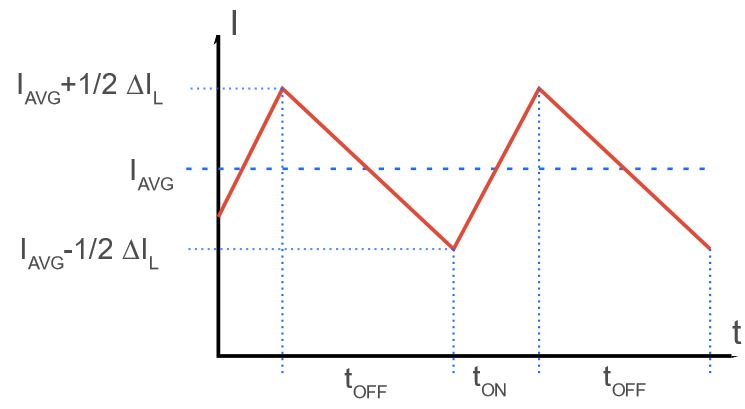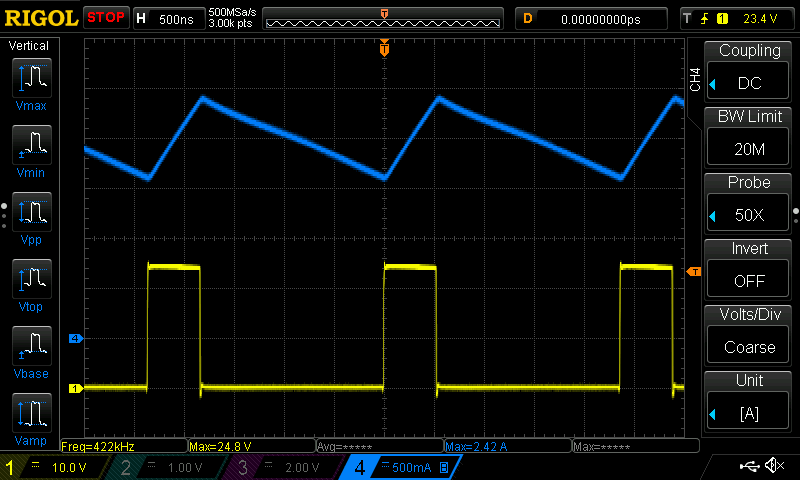## 饱和电流(ISAT)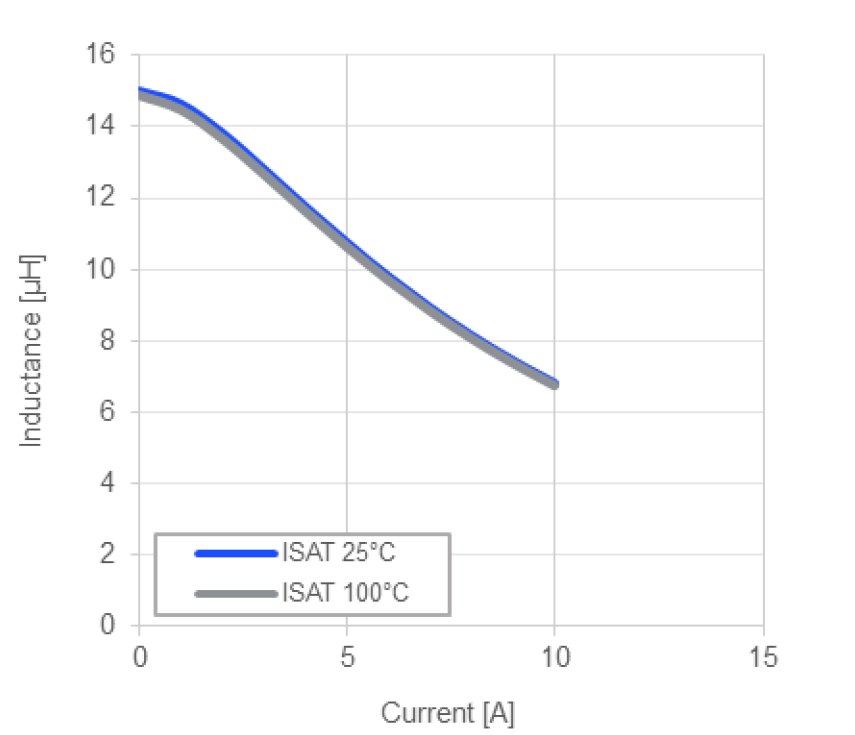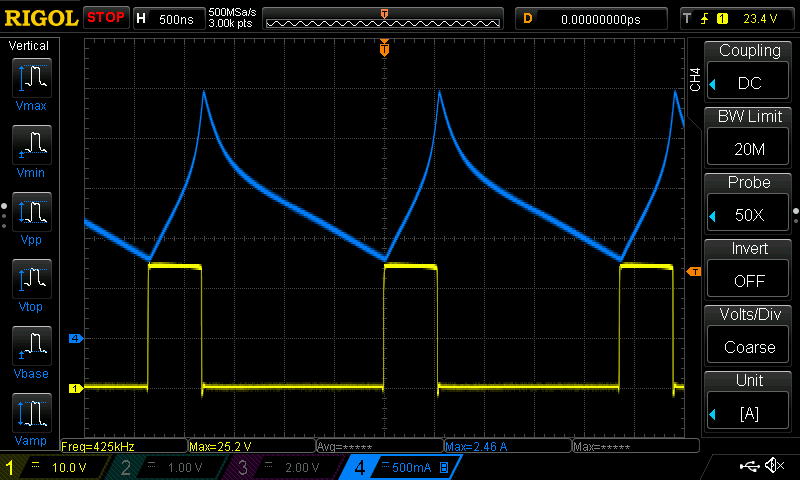## 额定电流(IR)和直流电阻(RDC)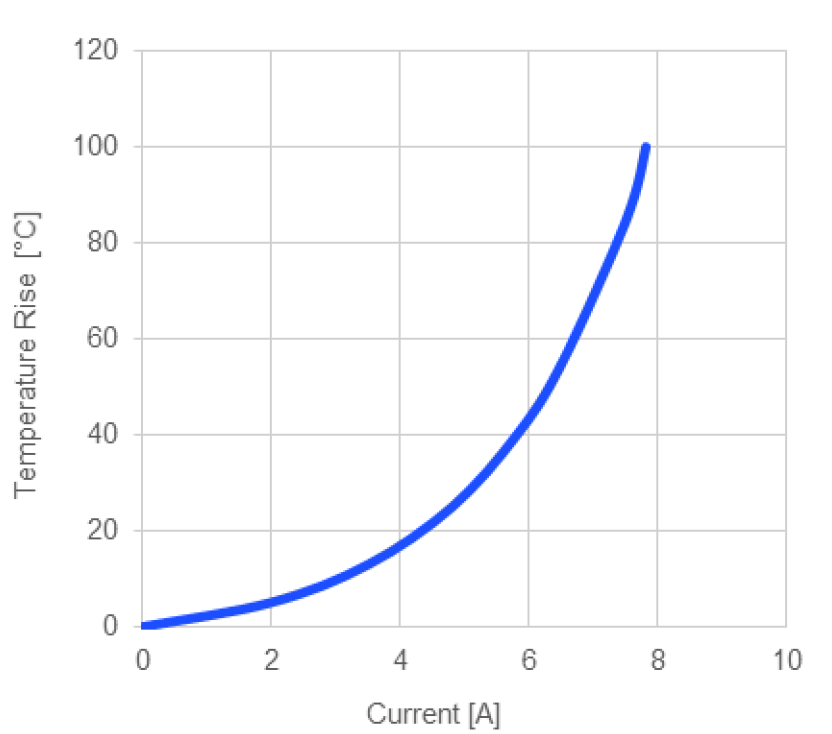## 匹配电感和降压稳压器以获得最佳效率

$$P_{LOSS} = I^2 \times R_{DC}$$